Chapter Notes: Linear Equations in Two Variables

# Linear Equations in Two Variables Chapter Notes - Mathematics (Maths) Class 9

## Introduction

We know that a linear equation in one variable x is an equation in the form of ax + b = 0, where a, b are real numbers such that a ≠ 0. The value of the variable which satisfies a given linear equation is known as its solution. The solution of a linear equation is also known as its root. If ax – b = 0 is a linear equation, then x = b/a  is its solution or root. The linear equation in one variable has a unique solution. Also, it gives a straight line when plotted on a graph.

Let us consider a simple example of a linear equation in one variable
(i) 2x = 8
Here, x = 8/2 = 4 (Unique solution)
If we plot the solution of this equation on the graph we get a straight  line.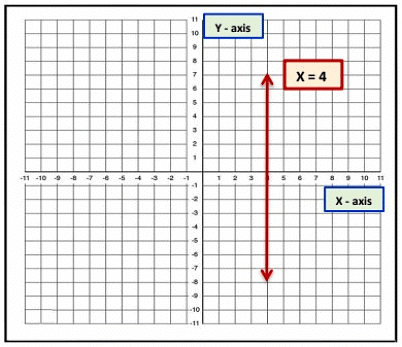In this chapter, we recall our previous knowledge and extend it to that of the linear equation in two variables.

Linear Equations
An equation in which the maximum power of the variable is one is called a linear equation.

Example: x – 2 = 5, x + y = 15 and 3x – 3y = 5 are some linear equations.

Linear equations can be used to solve real-life problems such as
(i) To know the cost of five pencils if the cost of one pencil is known.
(ii) Weather predictions
(iii) To express cricket score
(iv) To know how many chocolates and balloons we can buy for the money we have
(v) Government surveys.

(1) Linear Equation in one variable: The equation of the form ax + b = 0, where a and b are real numbers such that a ≠ 0 and x is a variable, is called a linear equation in one variable.
Example:

(i) 3x + 3 = 12,
(ii) t + 2t = 7 – t

(2) Linear Equation in two variables: An equation of the form ax + by + c = 0, where a, b and c are real numbers, such that, (a and b are not both zero), and x and y are the two variables, is called as a linear equation in two variables.
Example:

(i) 3x + 2y − 5 = 0,
(ii) x − 4 = √3y

Let us consider another example of linear equation in two variables,
One day, Richa and Pranita went to Mango orchard. They both started collecting mangoes, after two hours they got tired and sat under a tree. After some time they started counting the number of mangoes collected and found that they have collected 79 mangoes in 2 hours. If we have to represent a situation in the form of an equation it is written as
x + y = 79
Here,
x: Number of mangoes collected by Richa
y: Number of mangoes collected by Pranita
We don’t know how many mangoes are collected by each one of them i.e., there are two unknown quantities. Hence, we used x and y to denote them.
So, x + y = 79
This is the required equation.
The solution of a linear equation is not affected when:
(i) The same number is added (or subtracted) from both sides of the equation.
Example: (i) 4 + 2 = 2 × 3

When we subtract 5 from both sides of the given equation we get;
(4 + 2) − 5 = (2 × 3) − 5
6 − 5 = 6 − 5
1 = 1
LHS = RHS
Hence, we can conclude that the solution of a linear equation is not affected when the same number is subtracted from both sides of the equation.

Example: (ii) 4 + 2 = 2 × 3

Added 5 to both sides of given equation and we get;
(4 + 2) + 5 = (2 × 3) + 5
6 + 5 = 6 + 5
11 = 11
LHS = RHS
Hence, we can conclude that the solution of a linear equation is not affected when the same number is added to both sides of the equation.

(ii) The same non-zero number is multiplied or divided both sides of the equation.

Example: (i) 4 + 2 = 9 − 3

Multiplied by 5 to the both sides of given equation and we get; (4 + 2) × 5 = (9 − 3) × 5
⇒ 6 × 5 = 6 × 5
30 = 30
LHS = RHS
Hence, we can conclude that the solution of a linear equation is not affected when we multiply both sides of the equation by the same non-zero number.

Example:(ii) 4 + 2 = 9 − 3

Divide by 3 to both sides of the given equation and we get;
(4 + 2) / 3 =  (9 - 3) / 3
(6) / 3 = (6) / 3
2 = 2
LHS = RHS
Hence, we can conclude that the solution of a linear equation is not affected when we divide both sides of the equation by the same non- zero number.

Let’s solve some examples on Linear equations:
Example: Write each of the following equations in the form ax + by + c = 0 and indicate the values of a, b and c in each case.
(i) 3x + 4y = 7,
(ii) 2x – 8 = √3y,
(iii) x = 4y,
(iv) X/2 - Y/4 = 5

(i) 3x + 4y = 7

The above equation can be re-written as
3x + 4y − 7 = 0
On comparing with ax + by + c = 0, we get
a = 3, b = 4, and c = −7

(ii) 2x – 8 = √3y

The above equation can be re-written as
2x – √3y − 8 = 0
On comparing with ax + by + c = 0, we get
a = 2, b = √3 , and c = − 8

(iii) 2x = 4y

The above equation can be re-written as
2x – 4y = 0
There is no constant value in the given equation. So, we can write the equation as
2x − 4y + 0 = 0 ........ [Adding zero does not change the equation]
On comparing with ax + by + c = 0, we get
a = 2, b = − 4 , and c = 0

(iv) X/2 - Y/4 = 5

2X - Y / 4= 5
2x − y = 5 × 4
2x − y = 20
2x − y − 20 = 0
On comparing with ax + by + c = 0, we get
a = 2, b = −1 , and c = −20

Write each of the following as an equation in two variables x and y
(i) x = −5
(ii) 3x = 7
(iii) 7y = 2

(i) x = −5

x + 5 = 0
1. x + 0. y + 5 = 0

(ii) 3x = 7

3x − 7 = 0
3. x + 0. y − 7 = 0

(iii) 7y = 2

7y – 2 = 0
0. x + 7. y – 2 = 0

Example: The cost of one book is thrice the cost of a pencil. Write the linear equation in two variables to represent this statement.

Let the cost of a book be Rs. x and that of a pen to be Rs.y. Then according to the given statement, we have
x = 3y
[OR]
1. x – 3y + 0 = 0

Example: The bus fare is as follows: For the first kilometre the fare is Rs. 6 and for the subsequent distance it is Rs. 4 per km. Taking the distance covered as m km and total

fare is Rs. n. Write a linear equation for this information.

Total distance covered = m km
Fare for the first km = 6 Rs.
Fare for rest of the distance = Rs. (m - 1)4
We already know the total fare given is n
Total fare = [6 + (m - 1)4]
n = 6 + 4m – 4
n = 4m + 6 - 4
n = 4m + 2
4m – n + 2 = 0
This is the linear equation for this information.

Example: John and Jimmi are two friends they are studying in class 9. Together they contributed 8 dollars for flood victims. Write a linear equation that satisfies the given data. Also, draw a graph for that.

Let the amount that John and Jimmi contributed to be x and y respectively.
Amount contributed by John + Amount contributed by Jimmi = 8. So, x + y = 8
This is a linear equation that satisfies the data.
To draw a graph we need to find a x and y co-ordinate which satisfy the equation
x + y = 8 ..........(1)
Put y = 0 in the equation (1)
x + 0 = 8
x = 8
x + y = 8..........(1)
Put x = 0 in the equation (1)
0 + y = 8
y = 8
So, it can be observed that (8, 0), (0, 8) satisfy the equation 1.
Therefore these are the solution of the above equation.
The graph is constructed as follow: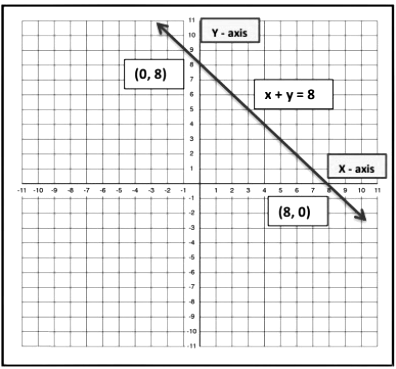### Solution of a Linear equation

“The values of variable involved in a linear equation which satisfy the equation i.e., the equation of LHS and RHS are equal, is called the solution of the linear equation.”

(i) Solution of Linear Equations in One Variable
“Any value of the variable that satisfies the given equation in x is called a solution or roots of the equation. We know the Linear equation in one variable has a unique solution.
Example:
(i) 3x + 3 = 12

3x = 12 – 3
3x = 9
x = 9/3
x = 3 is a solution of the given equation, which is unique.

(ii) 2x – 7 = 0

i. e. , 2x = 7
x = 7/2 is a solution of the given equation, which is unique.

(ii) Solution of Linear Equations in two Variables
There are two variables in the equation, a solution means a pair of values, one for x and one for y which satisfy the given equation. A linear equation in two variables has infinitely many solutions.
Example:

(i) x + 3y = 5

LHS = x + 3y
If we put x = 2 and y = 1 in the LHS of the equation we get,
LHS = x + 2y = 3 + 3(1) = 5
Here, LHS = RHS
So, we can say that x = 2 and y = 1 is a solution of the equation x + 3y = 5

Let us consider one more value of x and y.

Putting x = 1 and y = 2 in the LHS of the equation we get,
LHS = x + 3y
LHS = x + 3y = 1 + 3(2) = 7
Now, LHS ≠ RHS
Therefore, we can say that x = 1 and y = 2 is not a solution of the equation x + 3y = 5.

(ii) Show that (x = 1, y = 1) is a solution of 3x + 2y − 5 = 0

If we put x = 1 and y = 1 in the given equation
We have,
LHS = 3(1) + 2(1) − 5 = 0
LHS = 3 + 2 − 5 = 0
LHS = 5 − 5 = 0 = RHS
So, x = 1, y = 1 is a solution of 3x + 2y − 5 = 0

Write Find the value of k in the following case, if x = 2, y = 1 is a solution of the equations:
(i) 3x + 2y = k

Put x = 2, y = 1,then
3(2) + 2(1) = k
6 + 2 = k
k = 8

(ii)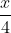+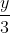= 5k

Put x = 2, y = 1, then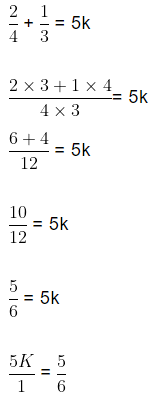k =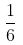For what value of k, the linear equation 2x + ky = 6 has x = 2 and y = 1 as its solution? If x = 4, then find the value of y.

The linear equation is 2x + ky = 6
At x = 2 and y = 1
2(2) + k(1) = 6
4 + k = 6
k = 6 – 4
k = 2 ... ... ... . (1)
If x = 4,then
2x + ky = 6
2(4) + 2y = 6 ....... [k = 2 from (1)]
8 + 2y = 6
2y = 6 – 8
2y = −2
∴ y = −1

### Equations of lines parallel to x-axis and y-axis

In the earlier topic, we have studied how to write the coordinates of a given point in the Cartesian plane.

Consider the points (2, 0), (-3, 0), (5, 0) etc. In the given points they co-ordinates are zero hence, they lie on the x-axis. Thus, the equation of x-axis in one variable is y = 0 and the equation of x-axis in two variables is 0.x + 1.y = 0. This equation shows that for each value of x, the corresponding value of y is zero.

The graphical representation of x-axis and y-axis as follow: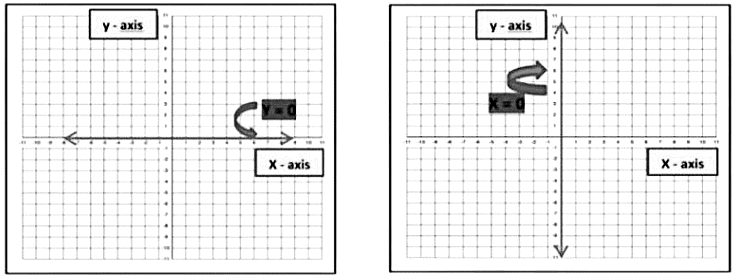Equation of Line parallel to y-axis:
Let x – 2 = 0 be an equation. Then,
(i) If it is treated as an equation in one variable x only, then it has a unique solution i.e., x = 2
(ii) If it is treated as an equation in two variables x and y, then it can be written as 1. x + 0. y – 2 = 0. This equation has infinitely many solutions of the form (2, a), Where a is any real number. Also, every point of the form (2, a) is a solution of this equation. Thus, the given equation represents the equation represents a line parallel to the y-axis.
So, the equation in two variables x – 2 = 0 is represented by line AB as shown in the figure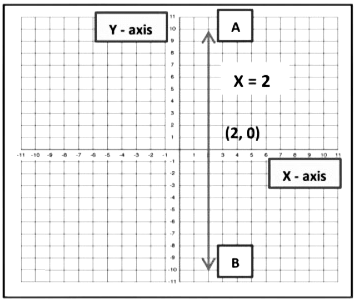Equation of Line parallel to x-axis:
Let y – 2 = 0 be an equation. Then,
(i) If it is treated as an equation in one variable y only, then it has a unique solution i.e., y = 2
(ii) If it is treated as an equation in two variables x and y, then it can be written as 0. x + 1. y – 2 = 0. This equation has infinitely many solutions of form (a, 2) where a, is any real number.
Also, every point of the form (a, 2) is a solution of this equation. Thus, the given equation represents the equation represents a line parallel to x-axis.
So, the equation in two variables y – 2 = 0 is represented by line AB as shown in the figure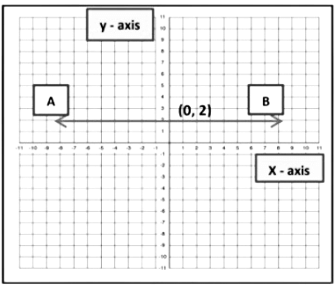Example: Solve the equation 2y – 3 = 12 – y. Represent the solution
(i) in the number line
(ii) in the cartesian plane

The given equation is 2y – 3 = 12 – y
2y + y = 12 + 3
3y = 15
y = 15/3
y = 5
(i) If we treated y = 5 as an equation in one variable only, then it has a unique solution y = 5. So, it is a point on the number line.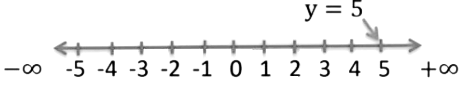(ii) If we treated y = 5 as an equation in two variables, then it can be written as 0. x + 1. y = 5 it is represented by a line. Here all the values of x are always zero. Because 0. x = 0. However, y must satisfy the equation y = 5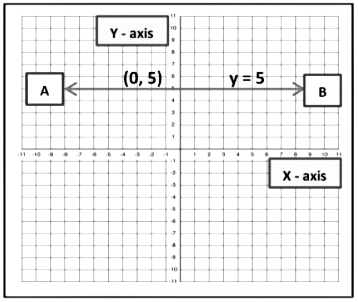The document Linear Equations in Two Variables Chapter Notes | Mathematics (Maths) Class 9 is a part of the Class 9 Course Mathematics (Maths) Class 9.
All you need of Class 9 at this link: Class 9

## FAQs on Linear Equations in Two Variables Chapter Notes - Mathematics (Maths) Class 9

 1. What is a linear equation in two variables?Ans. A linear equation in two variables is an equation that can be written in the form ax + by = c, where a, b, and c are constants and x and y are variables.
 2. How do you graph a linear equation in two variables?Ans. To graph a linear equation in two variables, we can use the slope-intercept form y = mx + b, where m is the slope and b is the y-intercept. We can plot the y-intercept on the coordinate plane and then use the slope to find additional points to draw a straight line connecting them.
 3. What is the solution to a system of linear equations in two variables?Ans. The solution to a system of linear equations in two variables is the ordered pair (x, y) that satisfies both equations simultaneously. In other words, it is the point where the lines representing the equations intersect on the coordinate plane.
 4. Can a system of linear equations in two variables have no solution?Ans. Yes, a system of linear equations in two variables can have no solution. This occurs when the lines representing the equations are parallel and do not intersect. In this case, there is no common point that satisfies both equations.
 5. How can we solve a system of linear equations in two variables algebraically?Ans. One common method to solve a system of linear equations in two variables algebraically is by substitution. This involves solving one equation for one variable and substituting that expression into the other equation. Another method is elimination, where we add or subtract the equations to eliminate one variable and solve for the remaining variable.

## Mathematics (Maths) Class 9

62 videos|426 docs|102 tests

## Mathematics (Maths) Class 9

62 videos|426 docs|102 tests
Signup to see your scores go up within 7 days! Learn & Practice with 1000+ FREE Notes, Videos & Tests.
10M+ students study on EduRev
Track your progress, build streaks, highlight & save important lessons and more!(Scan QR code)
Related Searches

,

,

,

,

,

,

,

,

,

,

,

,

,

,

,

,

,

,

,

,

,

;